Transient Response of Resistor-Capacitor (RC) and Resistor-Inductor (RL) Circuits
Time Constants

 Outline: The Transient Response of RC Circuits The Transient Response of RL Circuits The Complete Response Analysis Steps for finding the Complete Response of RC and RL Circuits Study Problems

The Transient Response of RC Circuits

The Transient Response (also known as the Natural Response) is the
way the circuit responds to energies stored in storage elements, such as
capacitors and inductors. If a capacitor has energy stored within it, then
that energy can be dissipated/absorbed by a resistor. How that energy is
dissipated is the Transient Response.

 In this circuit, there is a pulse, a resistor, and a capacitor. Assume here that the pulse goes from 10V down to 0V at t=0. Assume also that the circuit is in Steady State at t=0-. This implies that the capacitor is 'open' at t=0-. In order for KVL to be true at t=0- then the capacitor voltage must be 10V at t=0-. This is because there is no current in the circuit, therefore the voltage across the resistor is zero. Vc(0-) = Vc(0+) = 10V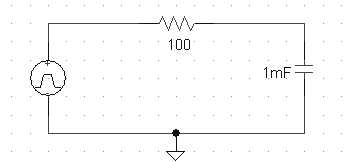Note that since the Transient Response is the circuit's response to energies stored in storage elements, we will 'kill' the pulse source. This leaves us with a simple Resitor-Capacitor circuit with an initial 10V on the capacitor at t=0+. Applying KCL to an RC circuit: Cdv/dt + V/R = 0 dv/dt + V/(RC) = 0 ∫dv/V = ∫-1/(RC) dt ln V = -t/(RC) + K ln V(t=0) = K ln Vo = K   ←Vo is the voltage on the cap at t=0+. lnV - ln Vo = -t/(RC) ln (V/Vo) = -t/(RC) V/Vo = e-t/(RC) V(t) = Vo e-t/(RC)   ←Vo = 10V in this example. Note that the speed at which the capacitor discharges from 10V to 0V is determined by the product R×C When t=RC, the voltage on the capacitor is Vo/e or 37% of it's initial value. We call RC the time constant and the symbol is τ For an RC circuit, τ=RC In this particular circuit τ = RC = 100Ω×1mF = 0.1 seconds This means it takes 0.1 seconds for the capacitor to discharge from 10V down to 3.7V. Looking at the response at the right, does this look about right?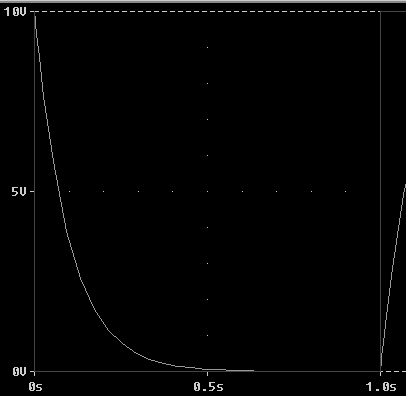Here is the same circuit as that above, except that the resistor value is doubled. This means that τ is also doubled. τ = RC = 200Ω×1mF = 0.2 seconds This circuit is twice as slow as the last circuit.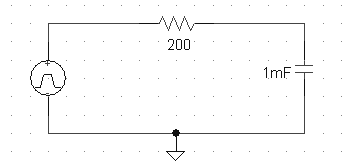Compare this response to the last one. Does it appear that it takes twice as long for the capacitor to discharge?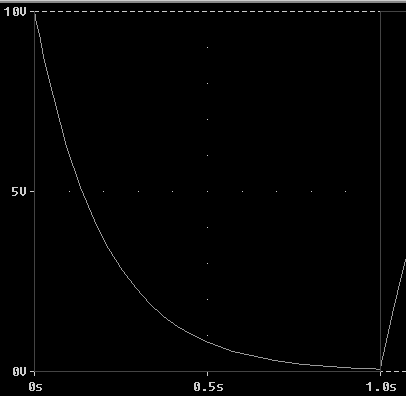Finding the Time Constant τ: Using an oscilloscope or P-Spice, we can calculate τ by inspecting the response curve. Locate the place where V = 37% × Vo. Then find the time at which this occurs. This time is the time constant, τ.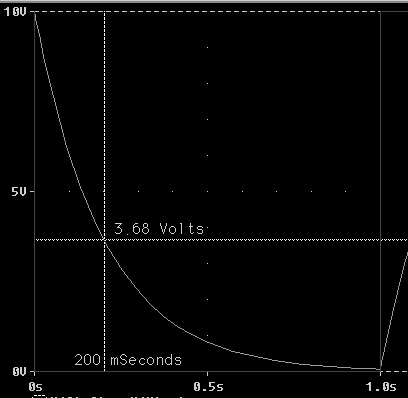The Time Constant  τ = RC for a simple RC-circuit.
The bigger τ is the longer it takes for the circuit to discharge.
The smaller τ is the faster the response.

τ is the time needed for the Transient Response to decay by a factor of 1/e.

## Study Problems

After clicking on the following link enter 7-1 for the problem and 1 for the step:
Study Problem 7-1

Top of Page

The Transient Response of RL Circuits

The Transient Response (also known as the Natural Response) is the
way the circuit responds to energies stored in storage elements, such as
capacitors and inductors. If an inductor has energy stored within it, then
that energy can be dissipated/absorbed by a resistor. How that energy is
dissipated is the Transient Response.

 In this circuit, there is a pulse, a resistor, and an inductor. Assume here that the pulse goes from -10V to 0V at t=0. Assume also that the circuit is in Steady State at t=0-. This implies that the inductor is a 'short' at t=0-. In order for KCL to be true at t=0- the inductor current must be -1A at t=0-. IL(0-) = IL(0+) = -1A Consider the circuit at t=0+, the voltage across the pulse is zero but since IL(0+) = -1A then VR = -10V. Therefore for KVL to be true VL = +10V. Therefore VL = +10V is the initial voltage across the inductor.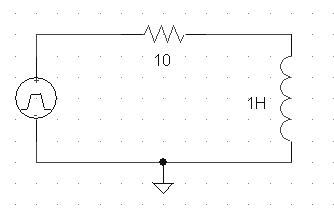Note that since the Transient Response is the circuit's response to energies stored in storage elements, we will 'kill' the pulse source. This leaves us with a simple Resitor-Inductor circuit with an initial -10A going through the inductor at t=0+. Applying KVL to an RL circuit: iR + Ldi/dt = 0 iR/L + di/dt = 0 -iR/L = di/dt -R/L dt = di/i ∫-R/L dt = ∫di/i -Rt/L + K = ln i K = ln i(t=0) K = ln io -Rt/L = ln i - K -Rt/L = ln i - ln io -Rt/L = ln(i/io) i/io = e-Rt/L i(t) = ioe-Rt/L    ←io in this case is -1A Since the plot on the right is for voltage we will find VL using VL = Ldi/dt VL = (1H) d[ioe-Rt/L]/dt = (1H) (-10) ioe-Rt/L VL = -10 e-Rt/L When t=L/R, the voltage on the inductor is Vo/e or 37% of it's initial value. We call L/R the time constant and again the symbol is τ For an RL circuit, τ=L/R In this particular circuit τ = L/R = 1H/10Ω = 0.1 seconds This means it takes 0.1 seconds for the inductor to go from 10V down to 3.7V. Looking at the response at the right, does this look about right?Here is the same circuit as that above, except that the resistor value is halved. This means that τ is doubled. τ = L/R = 1H/5Ω = 0.2 seconds This circuit is twice as slow as the last circuit.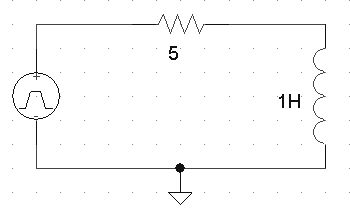Compare this response to the last one. Does it appear that it takes twice as long for the circuit to dissipate it's energy?Finding the Time Constant τ: Using an oscilloscope or P-Spice, we can calculate τ by inspecting the response curve. Locate the place where V = 37% × Vo. Then find the time at which this occurs. This time is the time constant, τ.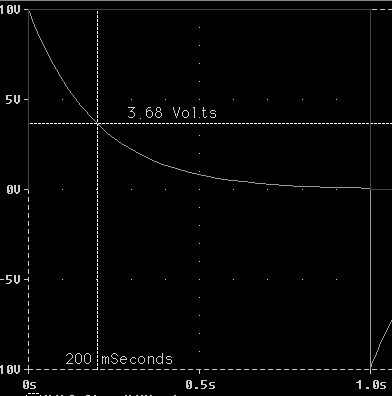The Time Constant  τ = L/R for a simple RL-circuit.
The bigger τ is the longer it takes for the circuit energy to discharge.
The smaller τ is the faster the response.

τ is the time needed for the Transient Response to decay by a factor of 1/e.

## Study Problems

After clicking on the following link enter 7-2 for the problem and 1 for the step:
Study Problem 7-2

Top of Page

The Complete Response

The Complete Response is the circuit's response to both
an independent source as well as energies stored in the circuit.

A circuit driven by an independent source is said to have
a forcing function.
Vcomplete response = Vnatural + Vforced

 Here is an RC Circuit with a Forcing Function: Assume the source is a pulse which goes from 0V to 10V at t=0. If we assume steady state at t=0-, then there is no initial energy stored in the circuit. Intuitively we know that the capacitor is going to charge up to 10V. When the capacitor gets to 10V then the circuit is again at steady state. The pulse is forcing the capacitor to 10V, thus the 10V on the capacitor is called the forced response. The time it takes the capacitor to charge up to 10V is determined by the time constant. The response of getting to 10V is the transient response.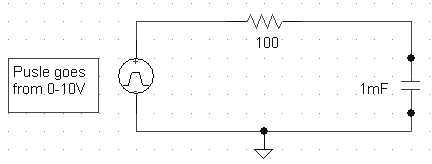Now we will find the Complete Response for V across the capacitor. This equation will match the curve shown at the right. From the last section we know that the transient response for an RC circuit is: V(t) = Vo e-t/(RC) = A e-t/(RC) Note that A is just some constant. We also know from inspection that eventually the capacitor will charge up to 10V. Now putting the transient and forced responses together we get: Vcomplete = A e-t/(RC) + Vforced Vcomplete = A e-t/(RC) + 10V Now we need to find A such that the equation equals Vo at t=0. In other words, the equation must satisfy the initial condition. V(t=0) = 0, therefore: 0 = A e0 + 10V = A + 10V A = -10V Vcomplete = -10e-t/(RC) + 10V Vcomplete = -10e-10t + 10V Note that when t>>0, Vcomplete = 10V. This intuitively means that when the transient response is gone the forced response still remains.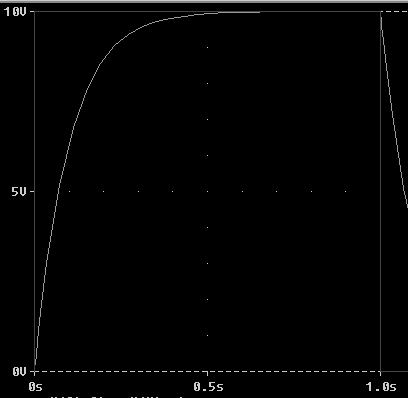In this circuit, the capacitor DOES NOT start at 0V. In other words the capacitor has a non-zero initial condition of 5V: Note that the left switch closes at the same time the right switch opens. Intuitively we can see that the capacitor is going to start at 5V and then charge up to 15V. For t<0 the 5V source is the forcing function and for t>0 the 15V source is the forcing function. Since this is an RC circuit with a forcing function, the response takes the following form: Vcomplete = A e-t/(RC) + Vforced By inspection we know that Vforced = 15V Vcomplete = A e-t/(RC) + 15V Now we need to find A such that the entire equation satisfies the value of V at t=0. V(t=0) = 5V = A e0 + 15V A = -10V Vcomplete = -10e-t/(RC) + 15 V Vcomplete = -10e-10t + 15 V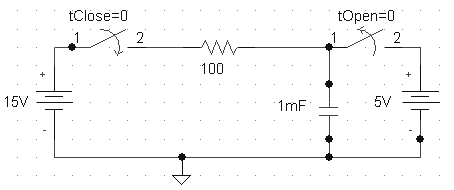Here is the Complete Response: Does this curve match the equation: Vcomplete = -10e-10t + 15 V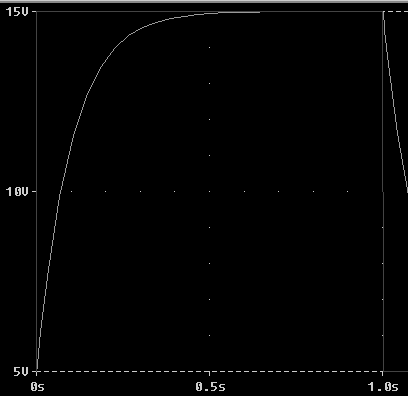Now let's find the voltage across the resistor for the RL circuit to the right. Note that the pulse goes from 5V to 15V at t=0. Assume that the circuit is in steady state at t=0-. At steady state inductors look like 'shorts' therefore the voltage across the resistor must be equal to the pulse voltage of 5V at t=0-. At t=0+ the voltage across the resistor is still 5V, but WHY????? Since the current in the inductor is continuous from 0- to 0+, and the current in the resistor is the same as the current in the inductor, and the voltage across the resistor is determined by its current, then we can say that if the resistor's current is continuous then the resistor's voltage must also be continuous. At t>>0 the voltage across the resistor is 15V. For t>0 we eventually reach steady state (as the transient response dies away), so we know that at t>>0 the inductor will look like a 'short'. Therefore the voltage across the resistor will equal the voltage of the pulse. Therefore we have both the initial condition and the forced response for the voltage across the resistor: Vo = 5V Vforced = 15V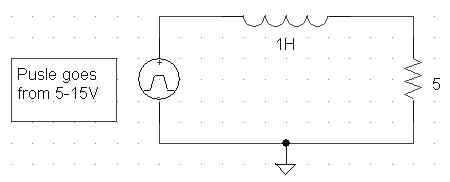Now we will find the Complete Response: Vcomplete = A e-t/τ + Vforced Vcomplete = A e-Rt/L + Vforced Vcomplete = A e-Rt/L + 15V To find A we must let t=0 and assign the equation to Vo: V(t=0) = 5V = A e0 + 15V = A + 15 A = -10 Vcomplete = -10 e-Rt/L + 15V Vcomplete = -10 e-Rt/L + 15V R/L = 5 Vcomplete = -10 e-5t + 15V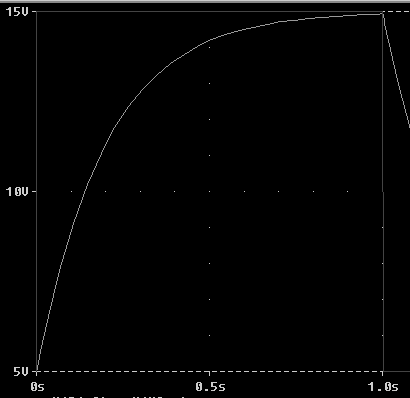Assume the pulse oscillates between 15V and 5V every 1 s. Consider the waveforms to the right. One is the pulse and the other is the voltage across the inductor. Note that as the transient response for VL dies away, the voltage across the inductor falls to zero. This is because the inductor acts like a 'short' in steady state conditions. However immediately after the switch moves a voltage is induced across the inductor. This voltage opposes the change that is occuring to the current in the circuit. Apply KVL to the circuit at t=0+ and t=1+ and see if you can get the 10V and -10V initial conditions for VL.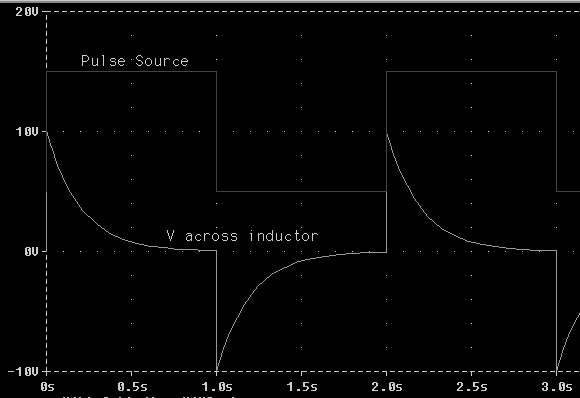The Complete Response has two parts: The Transient Response
and the Forced Response:

Vcomplete response = Vtransient + Vforced

Vtransient is found by 'killing' the forcing function. If the
circuit is RC then τ=RC and if the circuit is RL then τ=L/R.

Vforced is found by assuming Steady State. In other words, we
put the forcing function back into the circuit and assume that the
Transient Response has died out.

Any constants are found by setting Vcomplete(t=0) = Initial Conditions (at t=0)

Top of Page

Analysis Steps for finding the Complete Response of RC and RL Circuits

Use these Steps when finding the Complete Response for a 1st-order Circuit:

• Step 1: First examine the switch to see if it is opening or closing and at what time.

• Step 2: Next draw the circuit right before the switch moves. You will probably assume
steady state at this time but not always. The problem needs to tell you to assume steady state.

• Step 3: Find all voltages and currents that can not change instantaneously when the switch moves.
In other words, Find voltages across all capacitors and currents through all inductors!

• Step 4: Now draw the circuit right after the switch moves. Label the circuit with all the
capacitor voltages and inductor currents you found in step 3.

• Step 5: Now you are ready to find your initial condition(s). Analyze the circuit to find the
initial condition(s) of what it is your solving for.

• Step 6: Next you will find the transient/natural reponse, or τ. To do this 'kill' all forcing functions.
Make all voltage sources 'shorts' and all current sources 'opens'. Remember that the transient response is the circuit's
response to energies stored in storage elements, so we need to remove forcing functions to find this.
Recall that every voltage and current will have the same τ value.
You now have Ae-t/τ for what your solving for.

• Step 7: Now we need to find the forced response.
The forced response is the state of the circuit after the switch has moved AND after
the transient response has died-off. To find the forced response assume Steady State, i,e, t>>>0.

Find the final resting value (forced response - VF) of whatever it is you are solving for.

• Step 8: You should now have an equation which looks like v(t) = Ae-t/τ + VF
or i(t) = Ae-t/τ + IF.    To find the unknown 'A' you will apply the initial condition to
this equation. Usually the initial condition is the value at t=0, so you will plug in t=0 to get the following:
I.C. = A + VF   or    I.C. = A + IF     You can now solve for A.

• Step 9: Plugging the value of A into:  v(t) = Ae-t/τ + VF, you now
know the voltage for all time greater than t=0 (assuming that the switch moved at t=0).

• Step 10:  Using your equation for v(t) or i(t), you can find other things (voltages, currents,
power, etc.) using KVL, KCL, and Ohm's Law.

Top of Page

Study Problems

The following problems will follow the steps above to find the Complete Response of
first order circuits.

## Study Problems

After clicking on the following link enter 7-3 for the problem and 1 for the step:
Study Problem 7-3
After clicking on the following link enter 7-4 for the problem and 1 for the step:
Study Problem 7-4

Top of Page

Back To Index